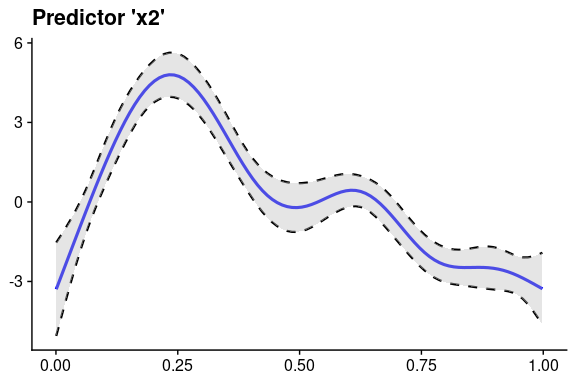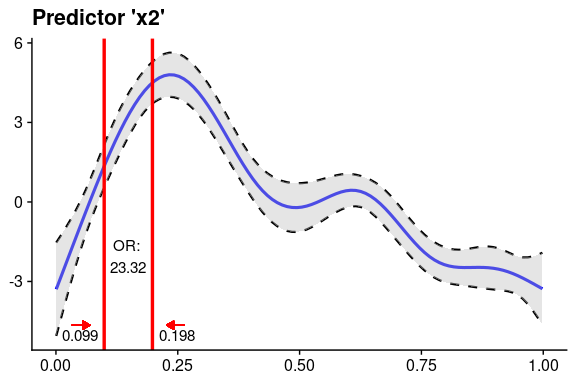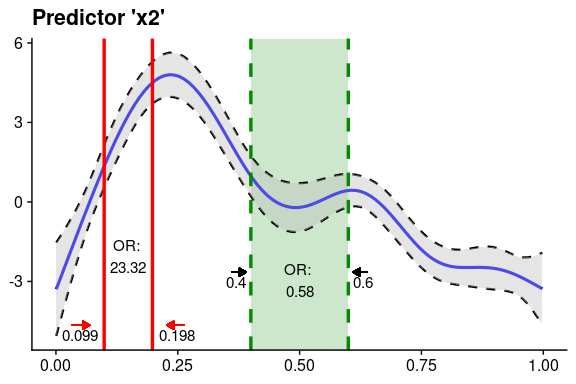# oddsratio tutorial

## Load example data & fit GAM model

Data source: ?mgcv::predict.gam

First, fit a simple GAM model.

library("oddsratio")

fit_gam <- mgcv::gam(y ~ s(x0) + s(I(x1^2)) + s(x2) + offset(x3) + x4,
data = data_gam)

## GAM example

### Calculating odds ratio for specific increment steps of a continuous variable

In this example we take predictor x2 (randomly chosen). We need to define start and stop values via argument values.

or_gam(data = data_gam, model = fit_gam, pred = "x2",
values = c(0.099, 0.198))
#> # A tibble: 1 x 6
#>   predictor value1 value2 oddsratio CI_low (2.5%) CI_high (97.5%)
#>   <chr>      <dbl>  <dbl>     <dbl>           <dbl>             <dbl>
#> 1 x2         0.099  0.198      23.3            23.3              23.3

In the plot it turns out that the odds of response y happening are 22 times higher when predictor x2 increases from 0.099 to 0.198 while holding all other predictors constant.

What is going on behind the scenes? Usually, this calculation is done by

1. setting all predictors to their mean value,
2. predict the response,
3. change the desired predictor to a new value and predict the response again. These steps result in two “log odds” values, which are transformed into “odds” afterwards. Finally, the odds ratio can be calculated from these two odds values.

### Calculating odds ratio for a level change of a nominal variable

If the predictor is an indicator/nominal/factor variable (i.e. consists of fixed levels) you can use the function in the same way by just putting in the respective factor levels:

or_gam(data = data_gam, model = fit_gam,
pred = "x4", values = c("A", "B"))
#> # A tibble: 1 x 6
#>   predictor value1 value2 oddsratio CI_low (2.5%) CI_high (97.5%)
#>   <chr>     <chr>  <chr>      <dbl>           <dbl>             <dbl>
#> 1 x4        A      B           1.38            1.33              1.42

Here, the change in odds of y happening if predictor x4 is changing from level A to B is rather small. In detail, an increase in odds of 37.8% is reported.

### Calculating odds ratio for percentage increments of a continuous predictor

To get an impression of odds ratio changes throughout the complete range of the smoothing function of the fitted GAM model for a specific predictor, you can calculate odds ratios based on percentage breaks of the predictors distribution.
Here we slice predictor x2 into 5 parts by taking the predictor values of every 20% increment step.

or_gam(data = data_gam, model = fit_gam, pred = "x2",
percentage = 20, slice = TRUE)
#> # A tibble: 5 x 8
#>   predictor value1 value2 perc1 perc2 oddsratio CI_low (2.5%)
#>   <chr>      <dbl>  <dbl> <chr> <chr>     <dbl>           <dbl>
#> 1 x2         0.001  0.2   0     20     2511.            1092.
#> 2 x2         0.2    0.4   20    40        0.03             0.03
#> 3 x2         0.4    0.599 40    60        0.580            0.56
#> 4 x2         0.599  0.799 60    80        0.06             0.06
#> 5 x2         0.799  0.998 80    100       0.41             0.75
#> # … with 1 more variable: CI_high (97.5%) <dbl>

We can see that there is a high odds ratio reported when increasing predictor x2 from 0.008 to 0.206 while all further predictor increases decrease the odds of response y happening substantially.

### Plot GAM(M) smoothing functions

Plotting smoother functions of GAM models is not very well supported in R. plot_gam() helps to simplify things:

plot_gam(fit_gam, pred = "x2", title = "Predictor 'x2'") +
theme_cowplot()You can further customize the look using other colors or line types. I highly recommend the themes from the cowplot in combination with oddsratio. However, you are of course free to go ahead with any other theme you prefer.

### Add odds ratio information into smoothing function plot

So now, we computed the odds ratios and created a plot of a GAM smoothing function. Why not combine both? This is what insert_or() is for. Its main arguments are

• ggplot plotting object containing the smooth function and
• a data frame returned from or_gam() containing information about the predictor and the respective values that should be inserted.
plot_object <- plot_gam(fit_gam, pred = "x2", title = "Predictor 'x2'")
or_object <- or_gam(data = data_gam, model = fit_gam,
pred = "x2", values = c(0.099, 0.198))

plot <- insert_or(plot_object, or_object, or_yloc = 3,
values_xloc = 0.05, arrow_length = 0.02,
arrow_col = "red")
plot +
theme_cowplot()The odds ratio information is always centered between the two vertical lines. Hence it only looks nice if the gap between the two chosen values (here 0.099 and 0.198) is large enough. If the smoothing line crosses your inserted text, you can just correct it adjusting or_yloc. This argument sets the y-location of the inserted odds ratio information.

Depending on the digits of your chosen values (here 3), you might also need to adjust the x-axis location of the two values so that they do not interfere with the vertical line.

Let’s do all of this by inserting another odds ratio result into this plot! This time we simply take the already produced plot as an input to insert_or() and use a new odds ratio object:

or_object2 <- or_gam(data = data_gam, model = fit_gam,
pred = "x2", values = c(0.4, 0.6))

insert_or(plot, or_object2, or_yloc = 2.1, values_yloc = 2,
line_col = "green4", text_col = "black",
rect_col = "green4", rect_alpha = 0.2,
line_alpha = 1, line_type = "dashed",
arrow_xloc_r = 0.01, arrow_xloc_l = -0.01,
arrow_length = 0.02, rect = TRUE) +
theme_cowplot()Using rect = TRUE, you can additionally highlight certain odds ratio intervals. Aesthetics like opacity or color are fully customizable.

## GLM example

Fit model.
Data source: https://stats.idre.ucla.edu/stat/data/binary.csv

fit_glm <- glm(admit ~ gre + gpa + rank, data = data_glm, family = "binomial")

### Calculate odds ratio for continuous predictors

For GLMs, the odds ratio calculation is simpler because odds ratio changes correspond to fixed predictor increases throughout the complete value range of each predictor.

Hence, function or_glm takes the increment steps of each predictor directly as an input in its parameter incr. To avoid false predictor/value assignments, the combinations need to be given in a list. Odds ratios of indicator variables are computed automatically and always refer to the base factor level.

The indicator predictor rank in this dataset has four levels. Subsequently, we will get three odds ratio outputs referring to the base factor level (here: rank1).

The output can be interpreted as follows: “Having rank2 instead of rank1 while holding all other values constant results in a decrease in odds of 49.1% (1-0.509)”.

or_glm(data = data_glm, model = fit_glm, incr = list(gre = 380, gpa = 5))
#> # A tibble: 5 x 5
#>   predictor oddsratio CI_low (2.5) CI_high (97.5) increment
#>   <chr>         <dbl>          <dbl>            <dbl> <chr>
#> 1 gre           2.36           1.05             5.40  380
#> 2 gpa          55.7            2.23          1511.    5
#> 3 rank2         0.509          0.272            0.945 Indicator variable
#> 4 rank3         0.262          0.132            0.512 Indicator variable
#> 5 rank4         0.212          0.091            0.471 Indicator variable

You can also set other confident intervals for GLM(M) models. The resulting tibble will automatically adjust the column names to the specified level.

or_glm(data = data_glm, model = fit_glm,
incr = list(gre = 380, gpa = 5), CI = 0.70)
#> # A tibble: 5 x 5
#>   predictor oddsratio CI_low (15) CI_high (85) increment
#>   <chr>         <dbl>         <dbl>          <dbl> <chr>
#> 1 gre           2.36          1.54           3.65  380
#> 2 gpa          55.7          10.1          315.    5
#> 3 rank2         0.509         0.366          0.706 Indicator variable
#> 4 rank3         0.262         0.183          0.374 Indicator variable
#> 5 rank4         0.212         0.136          0.325 Indicator variable